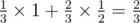# Magic Trick

Alex enjoys performing magic tricks. He has a trick that requires a deck of n cards. He has m identical decks of n different cards each, which have been mixed together. When Alex wishes to perform the trick, he grabs n cards at random and performs the trick with those. The resulting deck looks like a normal deck, but may have duplicates of some cards.

The trick itself is performed as follows: first Alex allows you to choose a random card from the deck. You memorize the card and put it back in the deck. Then Alex shuffles the deck, and pulls out a card. If the card matches the one you memorized, the trick is successful.

You don’t think Alex is a very good magician, and that he just pulls a card randomly from the deck. Determine the probability of the trick being successful if this is the case.

Input

First line of the input consists of two integers n and m (1 ≤ n, m ≤ 1000), separated by space — number of cards in each deck, and number of decks.

Output

On the only line of the output print one floating point number – probability of Alex successfully performing the trick. Relative or absolute error of your answer should not be higher than 10 - 6.

Examples

input

2 2

output

0.6666666666666666

input

4 4

output

0.4000000000000000

input

1 2

output

1.0000000000000000

Note

In the first sample, with probabilityAlex will perform the trick with two cards with the same value from two different decks. In this case the trick is guaranteed to succeed.

With the remainingprobability he took two different cards, and the probability of pulling off the trick is.

The resulting probability isSolution:

#include <cstring>
#include <vector>
#include <list>
#include <map>
#include <set>
#include <deque>
#include <stack>
#include <bitset>
#include <algorithm>
#include <functional>
#include <numeric>
#include <utility>
#include <sstream>
#include <iostream>
#include <iomanip>
#include <cstdio>
#include <cmath>
#include <cstdlib>
#include <ctime>
#include <memory.h>
#include <cassert>

using namespace std;

int main() {
int n, m;
scanf("%d %d", &n, &m);
if (n == 1) {
printf("1.0\n");
return 0;
}
if (m == 1) {
printf("%.17lf\n", (double)(1.0 / n));
return 0;
}
long double prob = 1.0;
for (int i = 0; i < m; i++) {
prob *= (m * n - n - i);
prob /= (m * n - i);
}
long double ans = 0.0;
long double sum = prob;
for (int k = 0; k <= m && k <= n; k++) {
ans += prob * k * k;
if (k == m || k == n) {
break;
}
prob *= (n - k);
prob /= (m * n - m - n + k + 1);
prob *= (m - k);
prob /= (k + 1);
sum += prob;
}
printf("%.17lf\n", (double)(ans / n));
return 0;
}